# [TOPSIC PG] How to exam

### Input value and output value

In TOPSIC, there are various patterns of input values ​​(called test cases) for each problem. It is required to write a program that gives correct answers to all test cases.

If there is the following problem:

[Problem] Find the value 10 times the given value.

Suppose the test case has the following three patterns.

• If 5 is given as the input value, 50 is presented as the output value.
• If 12 is given as the input value, 120 is presented as the output value.
• When 148 is given as an input value, 1480 is presented as an output value.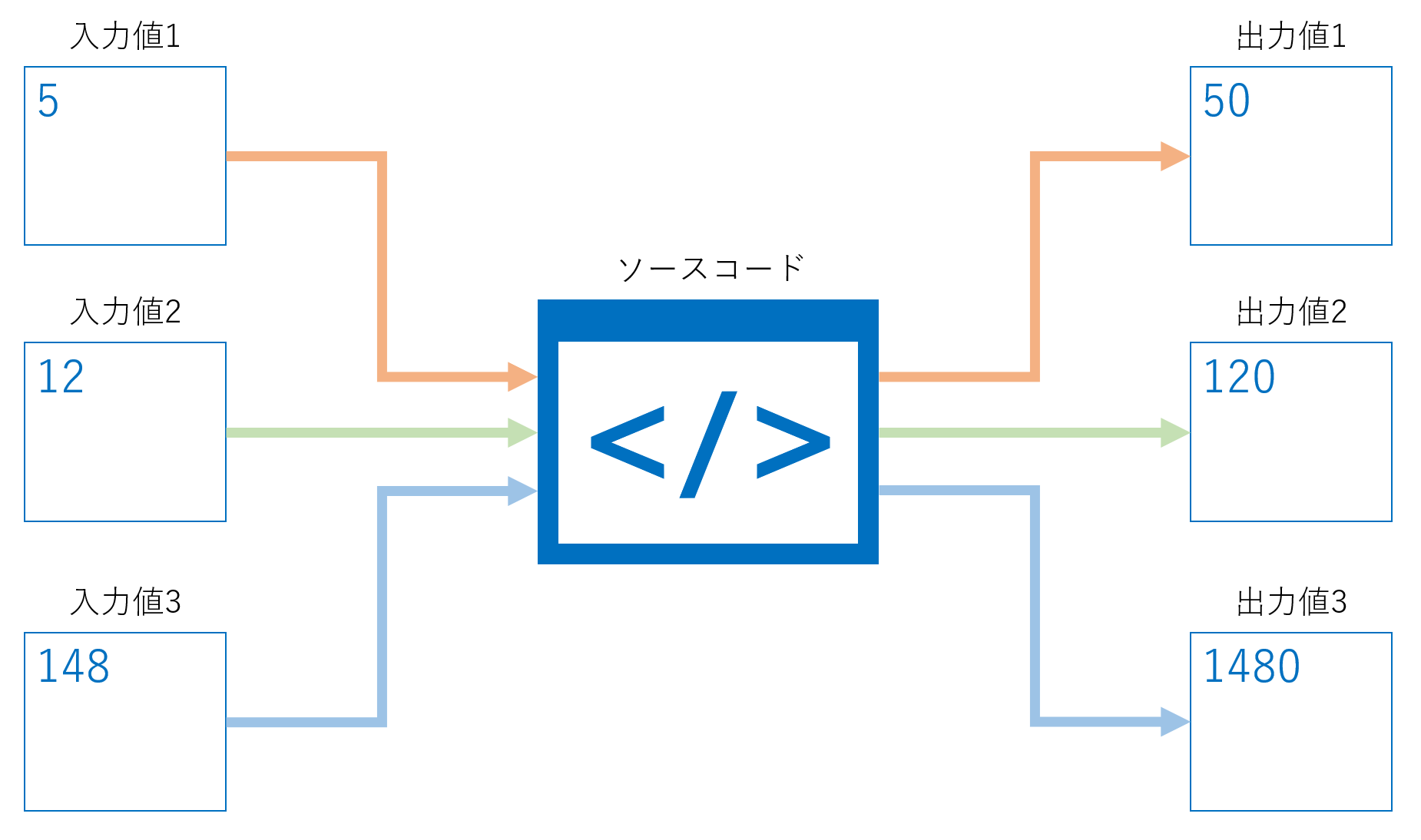As shown above, no matter what input value is given, the correct answer must be calculated and presented as the output value.

### Standard input and standard output

Standard input and standard output are used in TOPSIC.
Standard input / output is used for all problems. Please confirm in advance how to input and output.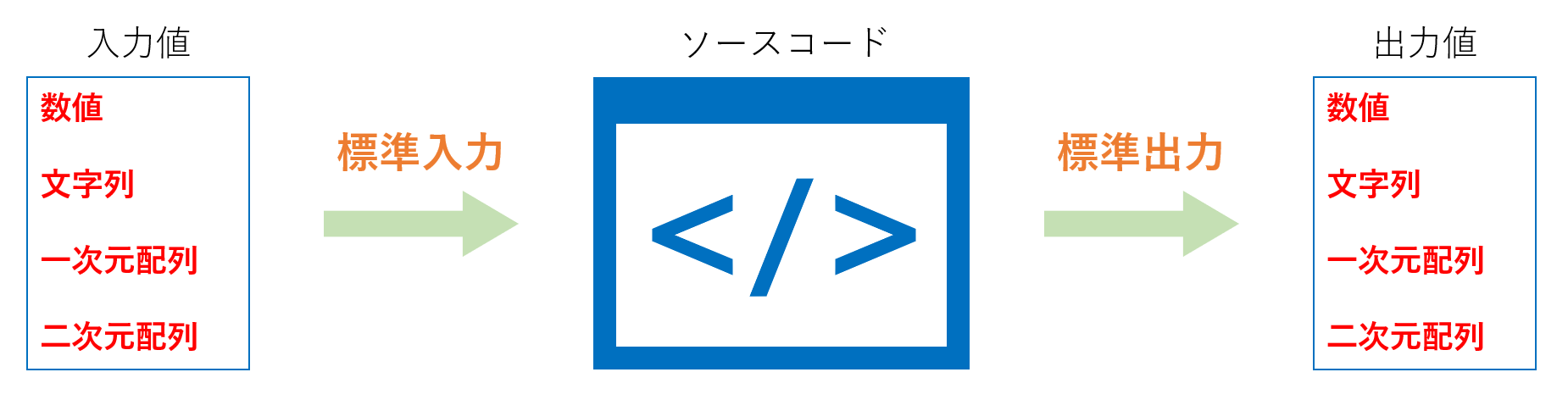##### Standard output

Use standard output to present the value calculated by the program as the answer.

The output format of the answer varies to the problems.Please answer in the output format suitable for the problem sentence, such as numerical value, character string, or array.

### Standard input / output method for each language

For information on how to write standard input and output in major languages, see the problem sentence of “Practice problems” in “How to Use”. The following languages ​​are listed.

• C
• C++
• Java
• C#
• PHP
• D
• Python3
• Perl
• Ruby
• Pascal
• JavaScript(Node.js)
• Scala
• COBOL - Free (OpenCOBOL 1.1.0)

## Take the TOPSIC

### Check the operation of the code editor

Let’s test the code. Display `Hello World!` using the TOPSIC code editor.

1. From “How to use” in the side menu, click “Custom Test”.
2. From “Language”, select the programming language. (Example: Java 8)
3. Write a code “Hello World!” in the “Source Code” area.
4. When you finish writing the code, click the “Execute Test Code” button at the bottom of the screen.
5. The custom test is executed. If the result is displayed in “Standard Output” it is successful.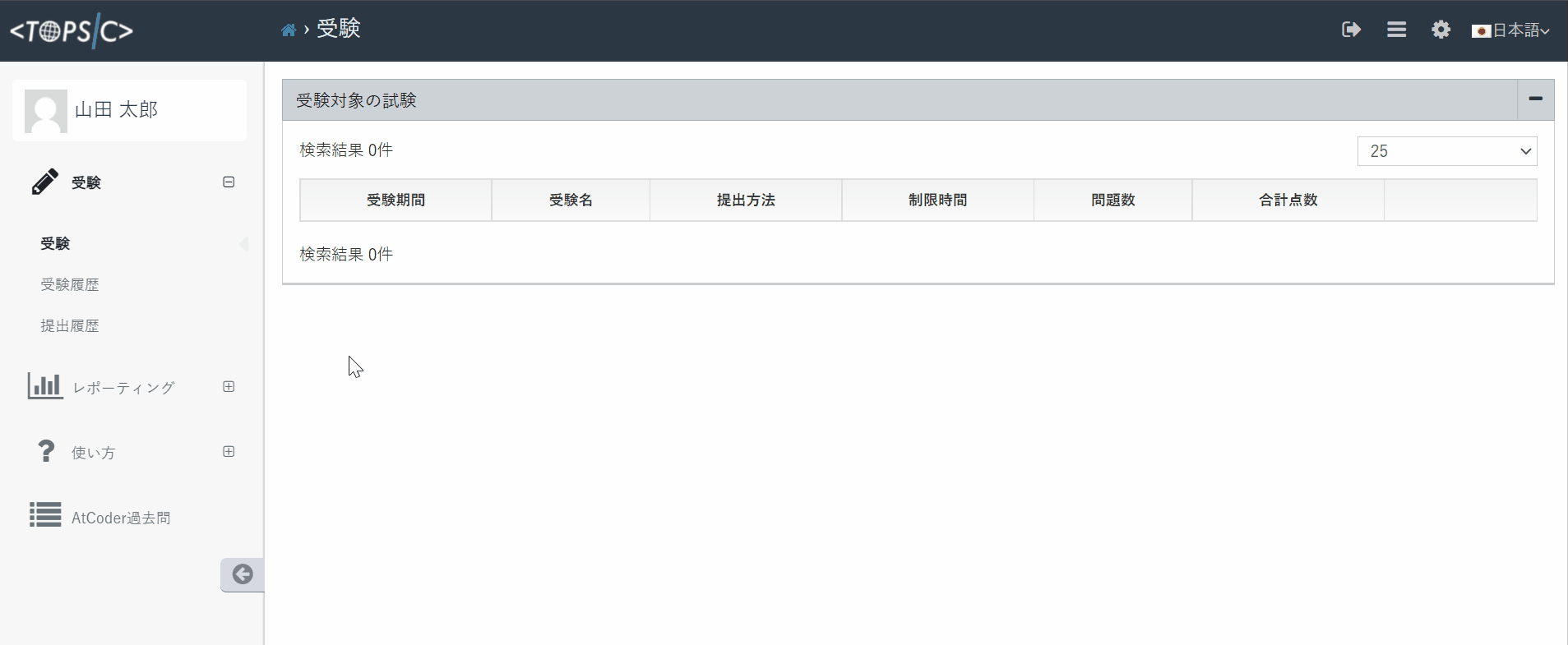### Take the practice problem

#### Start the exam

Let’s take the exercises.

1. From “How to use” in the side menu, click “Practice problems”.
2. Practice problems will be displayed, so click the “to Exam Detail” button. (Example: Hello TOPSIC)
3. Check the outline of the exam and notes.
4. After agreeing to the precautions, let’s start the test.
5. Once the exam starts, answer the questions within the time limit.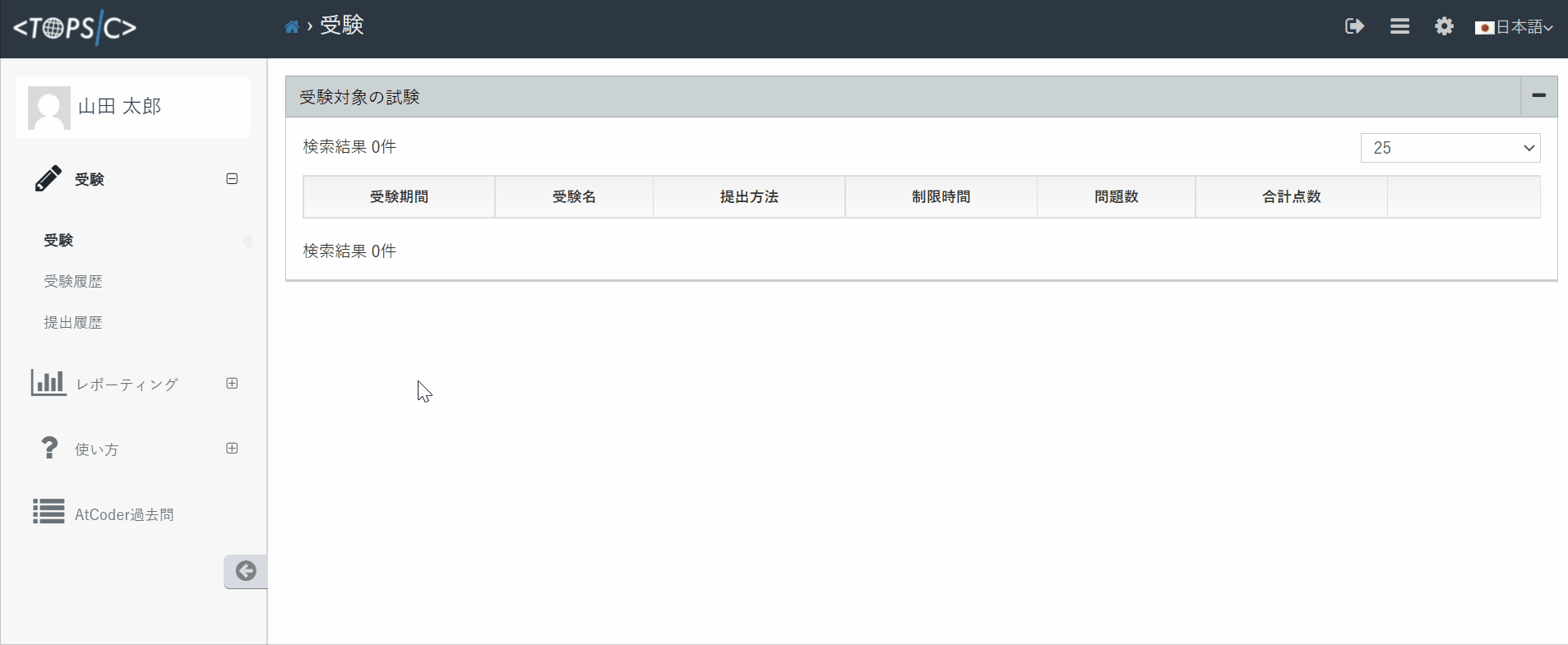#### Check the problems

At first, view the problems. Problems in this list are the problems of this exam.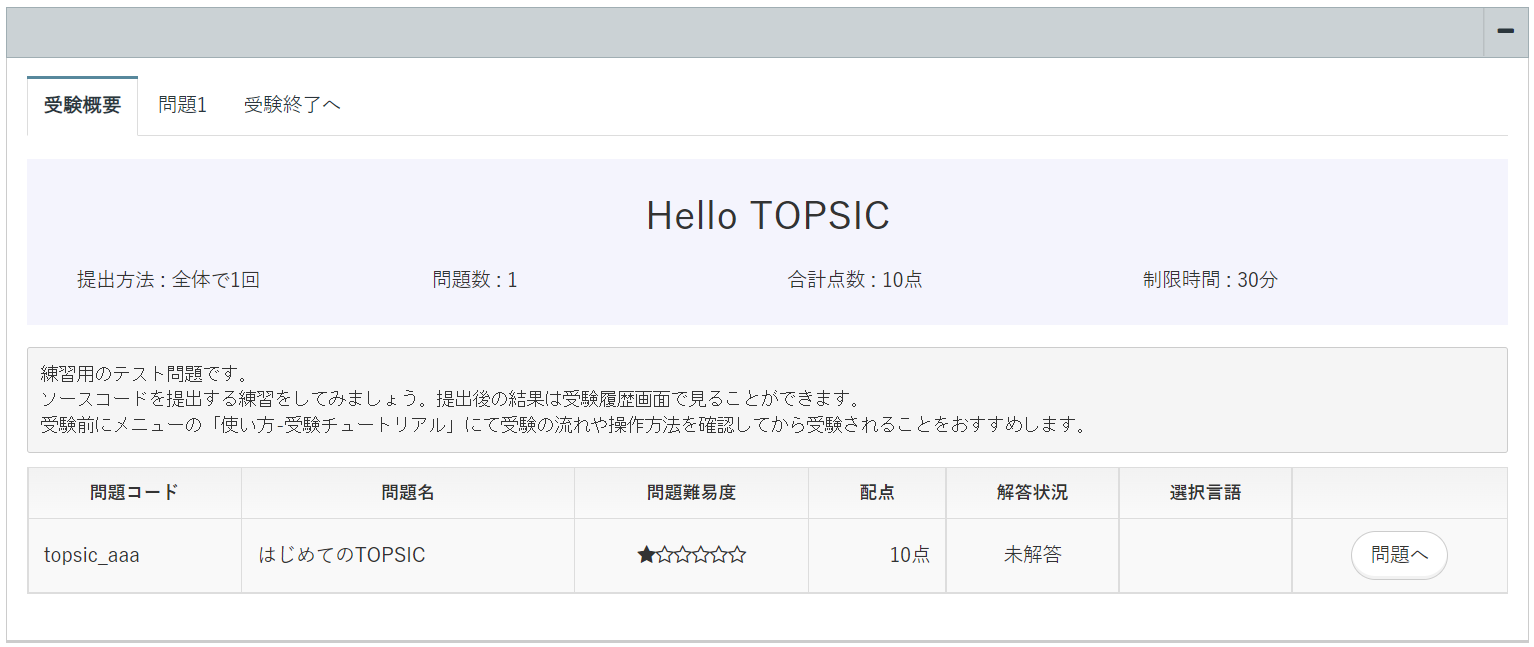You can move to the answer screen for each problem and the final submission screen with the tabs at the top of the screen. There is only one problem in the practice problem, but the reals exam may contain several problems. If there are multiple problems, it’s' better to solve as many problems as possible within time.

#### View the problems

To view the problem, click the tab or “to Problem” button. The practice problem title is “Welcome to TOPSIC”. Let’s think about how to solve the problem well.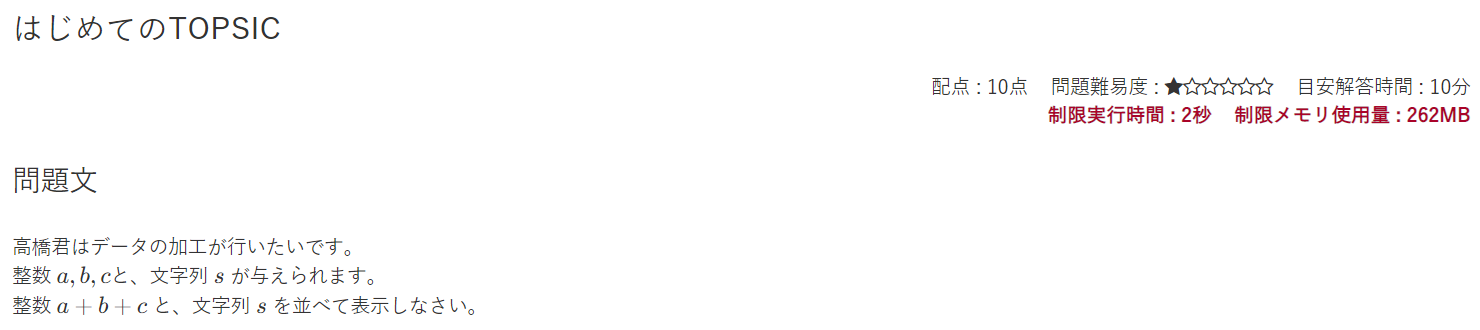To answer the problem correctly, it is necessary to write the code that fulfill the input format and the output format. You write Input in “Standard Input”. The code test output wiil be displayed in the “Standard Output”.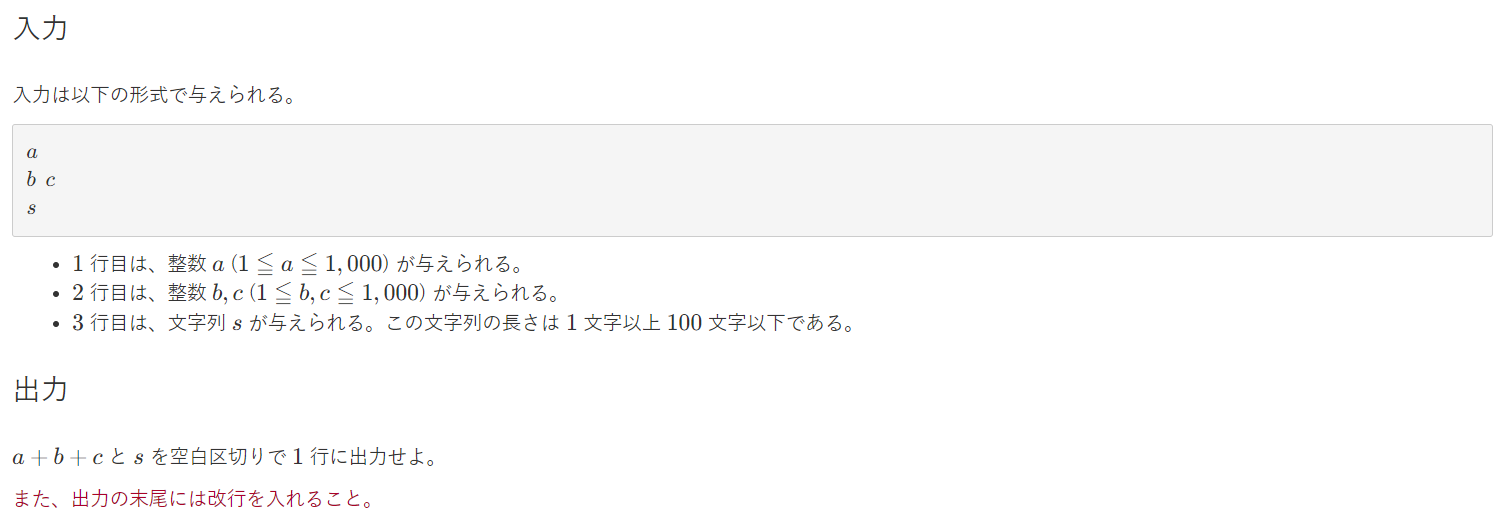In addition, input examples and output examples are shown in the problem. Write a code to get the output example using the input example argument.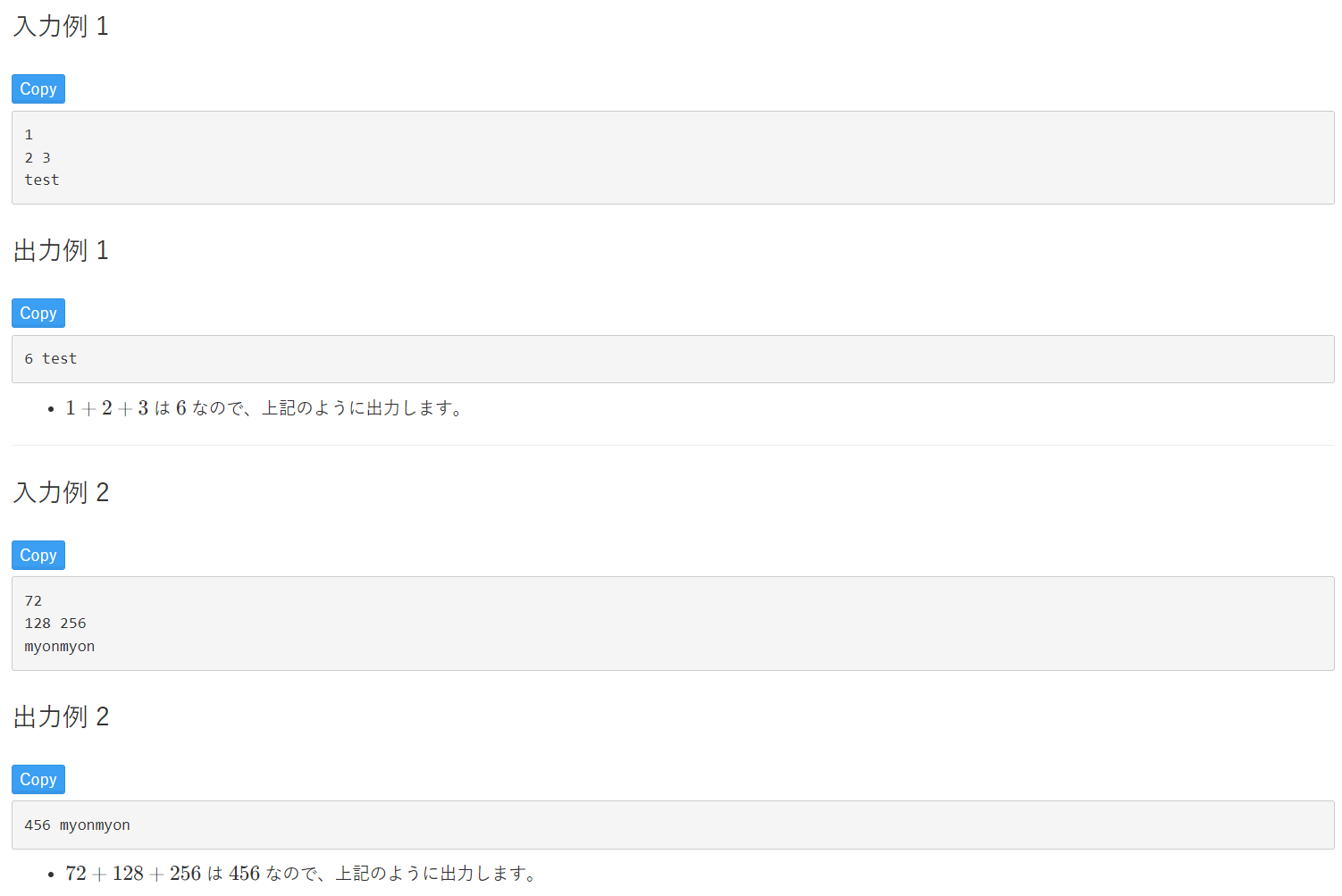The practice problem has notes and answer examples in the bottom of the problem.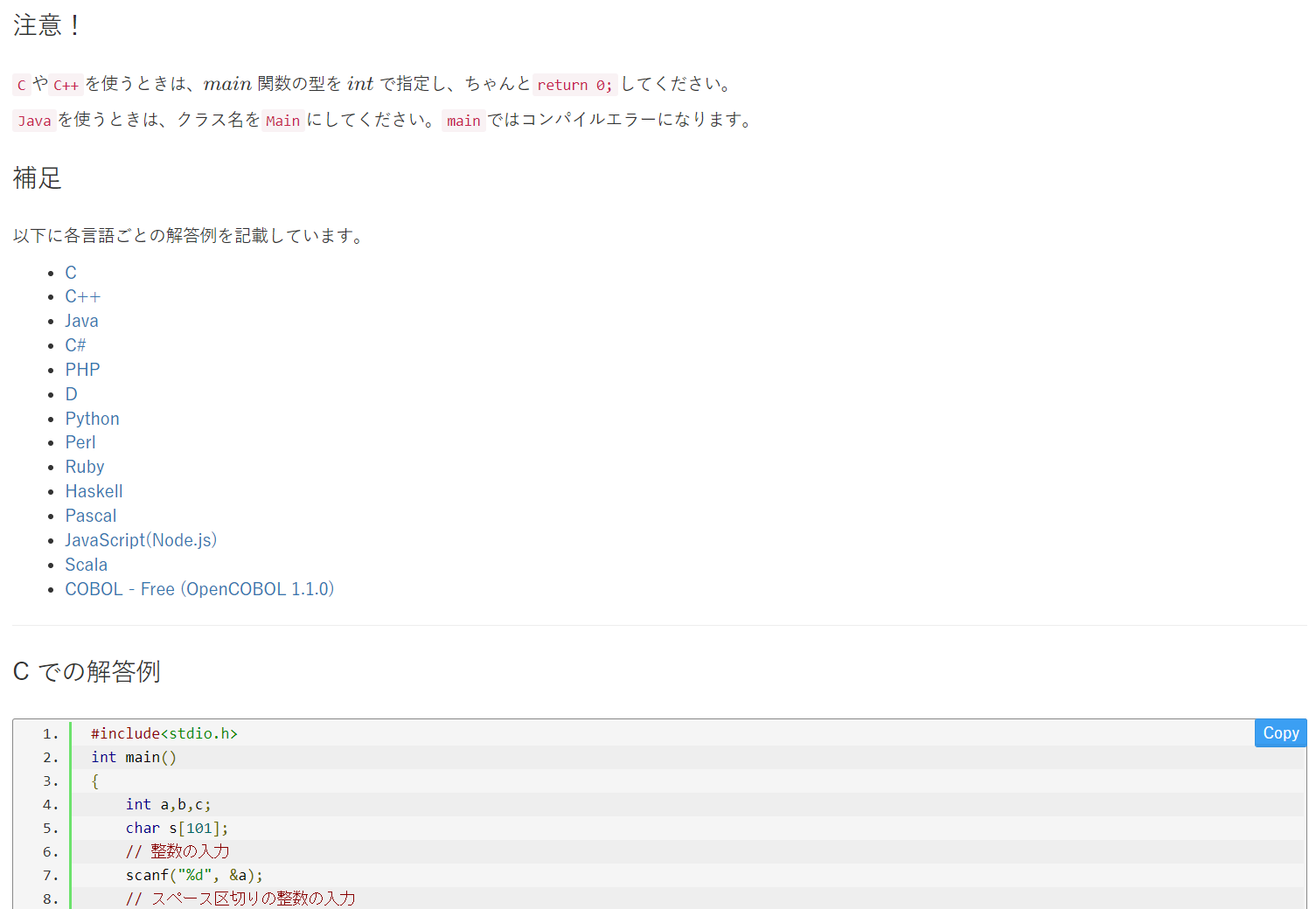Let’s read the problem and think about how to solve it. If you come up with a good way, let’s write the code in your own editor.

#### Test your code before submit

The system of TOPSIC is operating in Linux environment. Therefore, if you code in a different execution environment such as Windows, the line feed code or something else may differ. Before submitting, please check if the code test runs successfully.

1. Select the programming language. (Example: Java 8)
2. Paste your code to the source code area.
3. If there is no syntax error, write an example of problem input in the “Standard Input” field.
4. Click the “Execute Custom Test” button.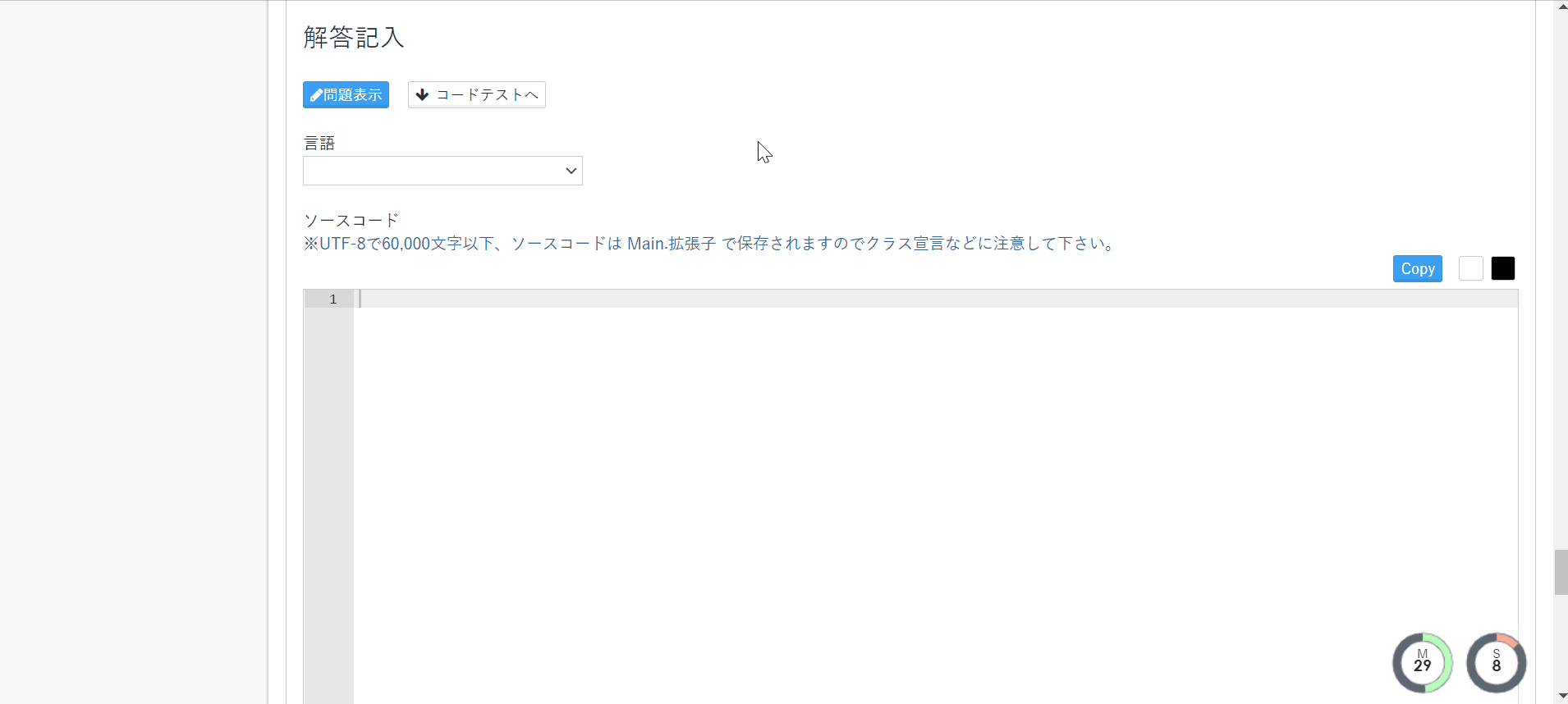A line feed code is included at the end of the sample input. If you copy by dragging, the line feed code may not be copied correctly, so please use the copy button when acquiring the sample input.

The test for the written code is executed. If the test code is executed successfully and your test output is same as the output example is returned, it is correct. If there is a problem with the code, a standard error is output. If the output result and the output example do not match, try again and again.

When the submission type is “One-shot”, click the “Save” button if there is no problem with your code.
By clicking the “Save” button, preparing the answer has completed.

When the submission type is “Unlimited”, there is the “Submit” button instead of the save button.
By clicking the “Submit” button, the answer will be submitted and redirected to the submissions page.

#### Check the score and submissions (only the submission type is unlimited)

When the submission type is “Unlimited”, you can check the current score and past submissions.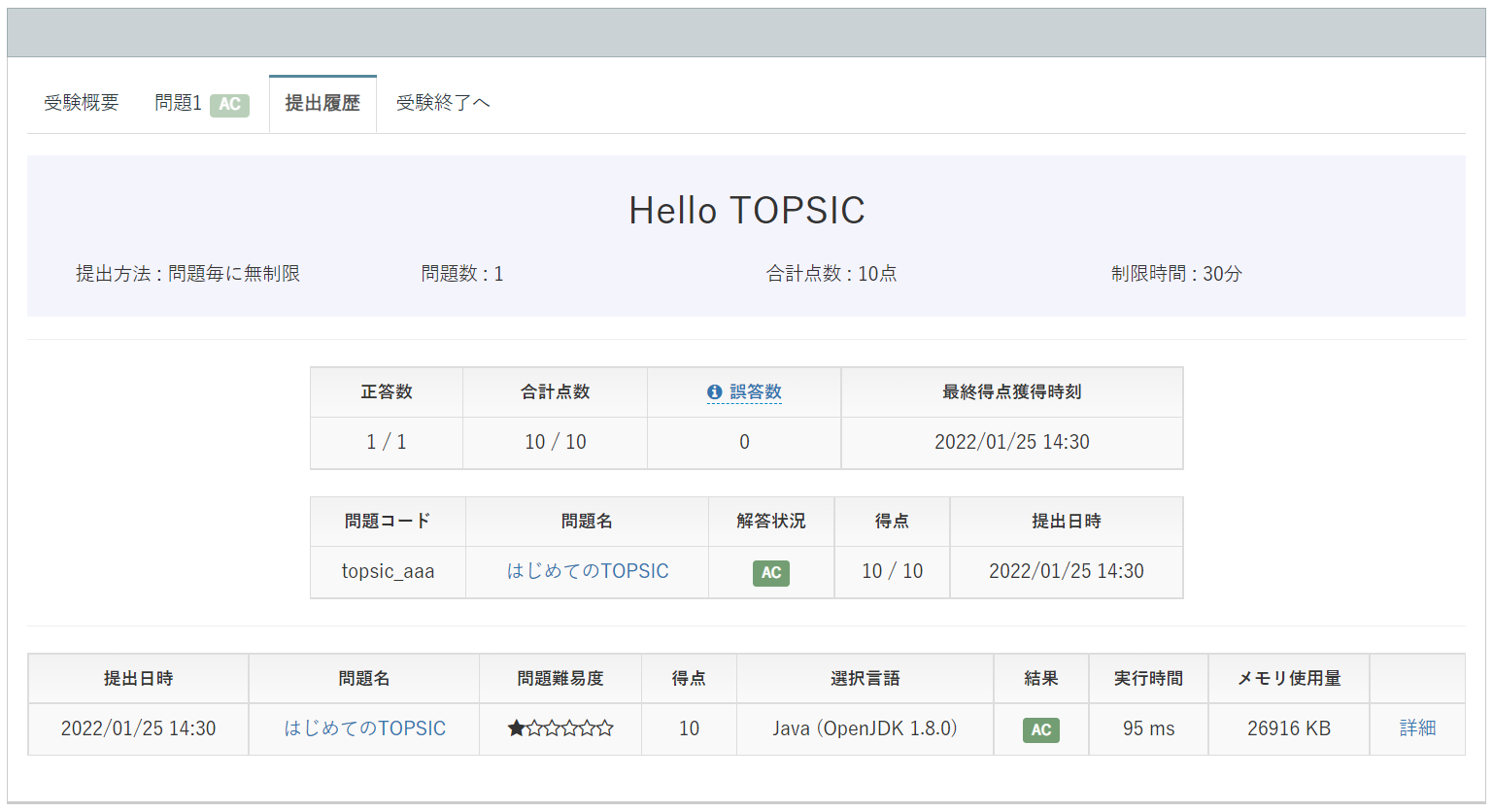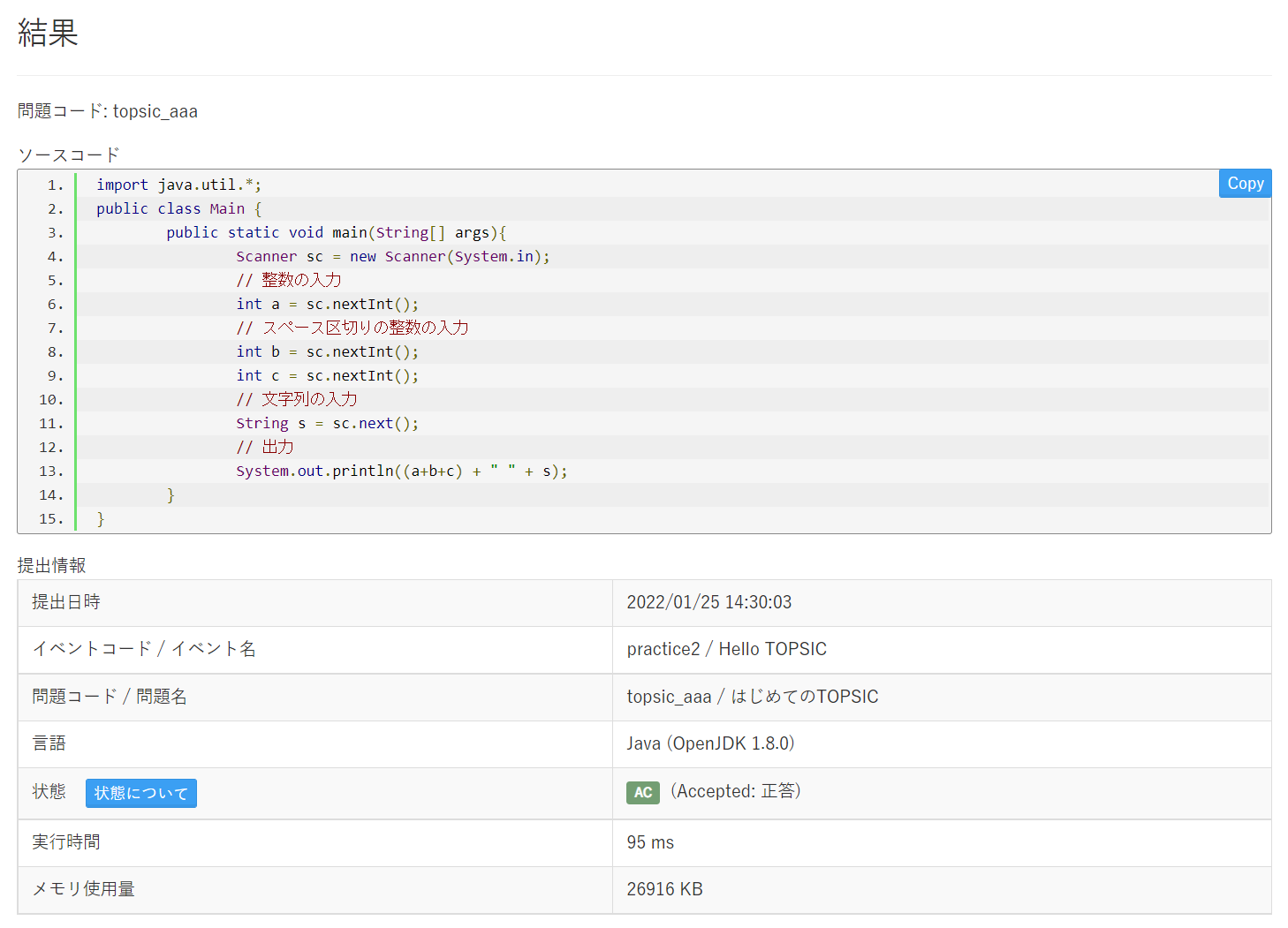#### Final submission

If you have other problems, repeat the above steps. After answering all the questions, proceed to final submission.
On the final submission screen, the problems that have been prepared the answer will be checked on “Answer status”.
Let’s submit all answers if you has finished. Click “Finish” button.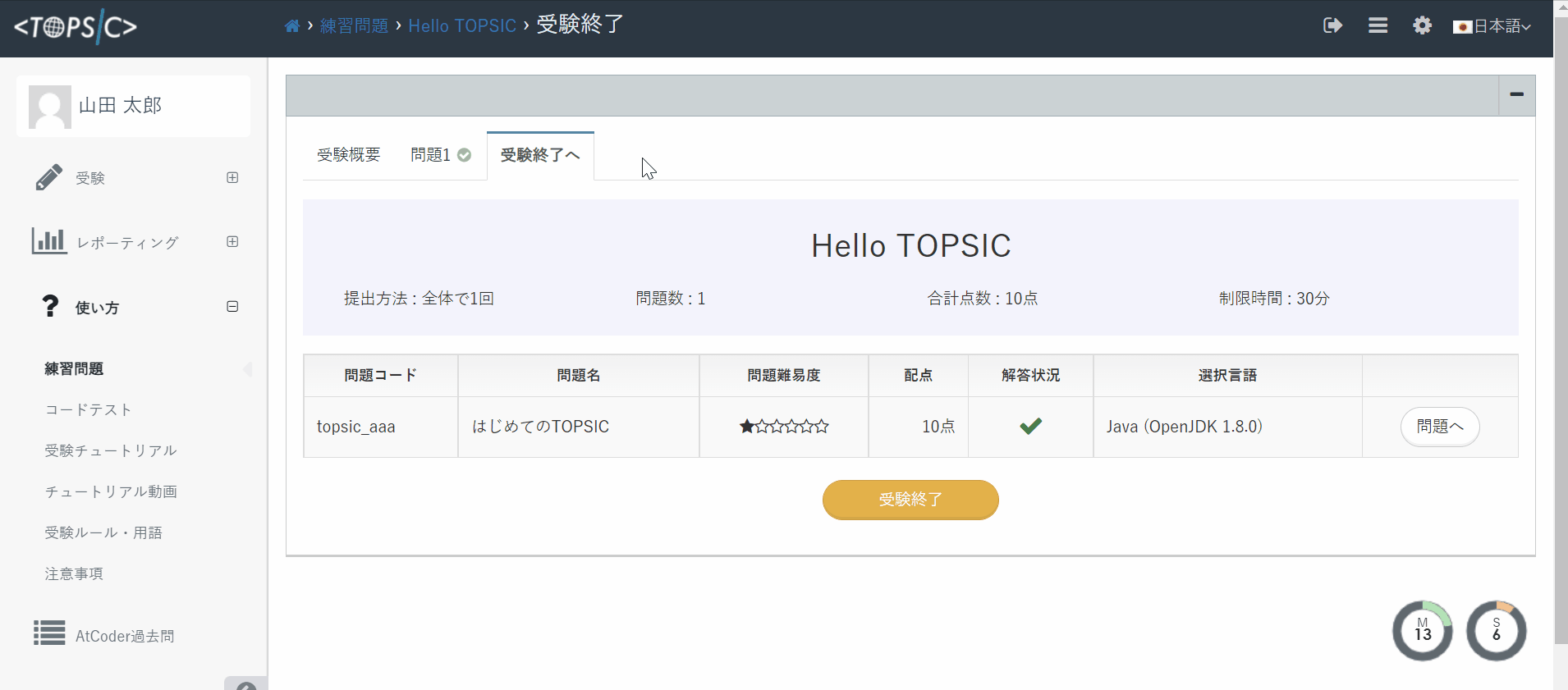The exam is over when the final submission. In the case of real exams, you cannot take the exam once you took.

If you click “Examination results” from the side menu, the taken exam will be displayed.

1. Click “Exam Name” to see the details of the result.
2. If you click “Detail”, the problem, your code, submission information and Compile message will be displayed.

If “Status” of submission information is AC (Accepted), it is correct. In addition to AC, there are many errors such as WA (Wrong Answer: Incorrect), CE (Compile Error), MLE (Memory Limited Error).

For other states, please check here.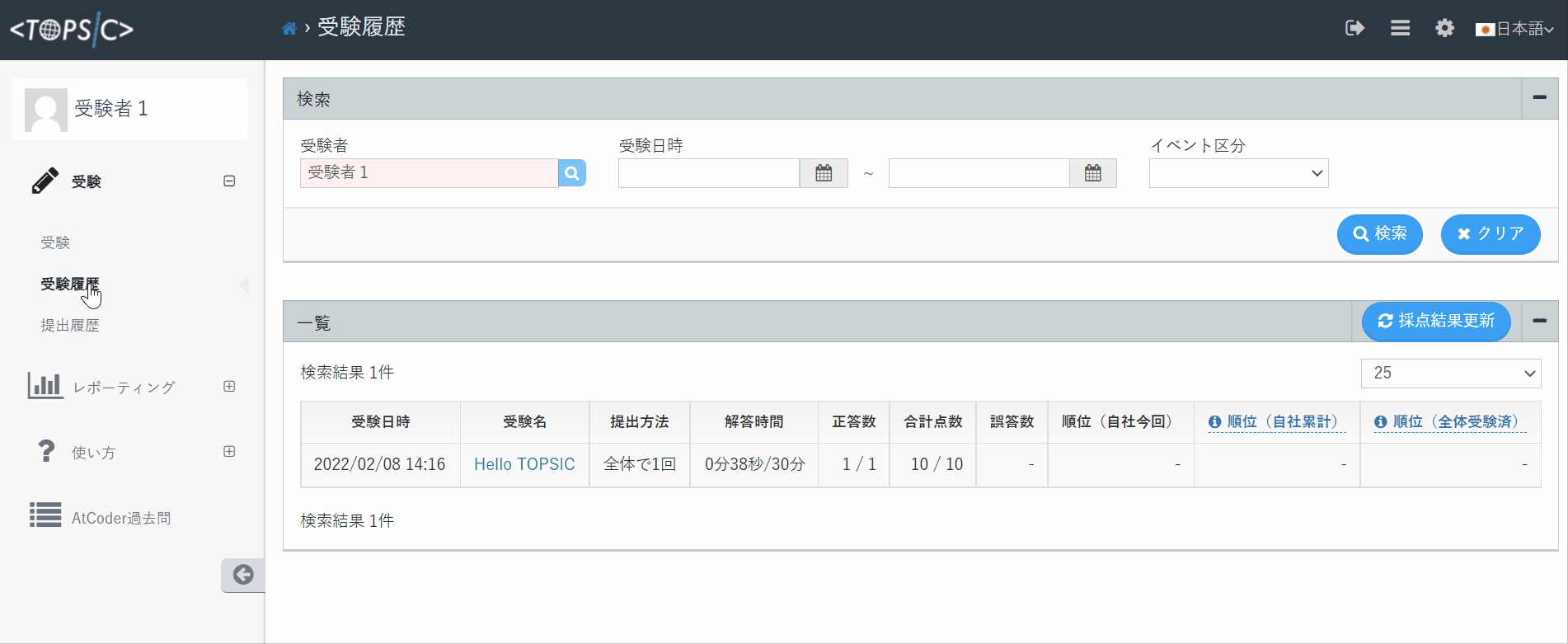## Take the real exams

In order to take the actual exam, click the “Examinations” in the side menu to display “to Exam Detail”. The flow after that will be the same as the practice problem.